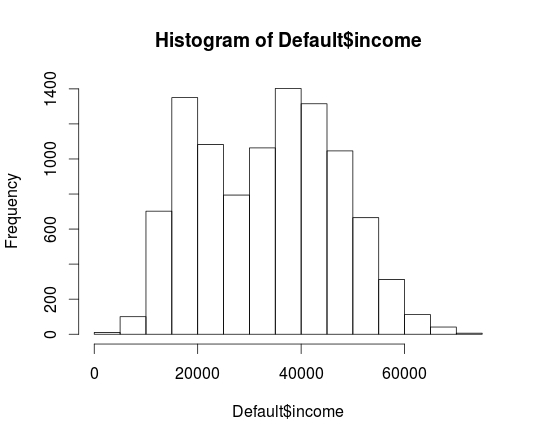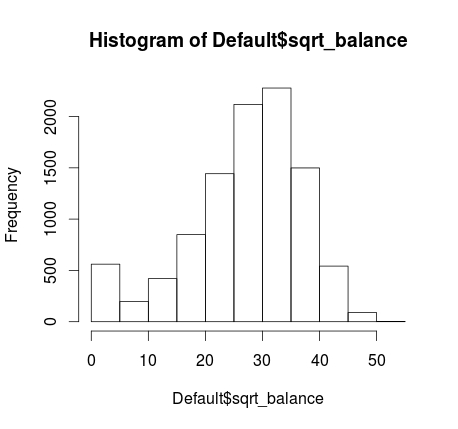# Logistic Regression in R

Logistic regression is used when the dependent variable is categorical with two choices. For example, if we want to predict whether someone will default on their loan. The dependent variable is categorical with two choices yes they default and no they do not.Interpreting the output of a logistic regression analysis can be tricky. Basically, you need to interpret the odds ratio. For example, if the results of a study say the odds of default are 40% higher when someone is unemployed it is an increase in the likelihood of something happening. This is different from the probability which is what we normally use. Odds can go from any value from negative infinity to positive infinity. Probability is constrained to be anywhere from 0-100%.

We will now take a look at a simple example of logistic regression in R. We want to calculate the odds of defaulting on a loan. The dependent variable is “default” which can be either yes or no. The independent variables are “student” which can be yes or no, “income” which how much the person made, and “balance” which is the amount remaining on their credit card.

Below is the coding for developing this model.

The first step is to load the “Default” dataset. This dataset is a part of the “ISLR” package. Below is the code to get started

``````library(ISLR)
data("Default")``````

It is always good to examine the data first before developing a model. We do this by using the ‘summary’ function as shown below.

``summary(Default)``
``````##  default    student       balance           income
##  No :9667   No :7056   Min.   :   0.0   Min.   :  772
##  Yes: 333   Yes:2944   1st Qu.: 481.7   1st Qu.:21340
##                        Median : 823.6   Median :34553
##                        Mean   : 835.4   Mean   :33517
##                        3rd Qu.:1166.3   3rd Qu.:43808
##                        Max.   :2654.3   Max.   :73554``````

We now need to check our two continuous variables “balance” and “income” to see if they are normally distributed. Below is the code followed by the histograms.

``hist(Default\$income)````hist(Default\$balance)``The ‘income’ variable looks fine but there appear to be some problems with ‘balance’ to deal with this we will perform a square root transformation on the ‘balance’ variable and then examine it again by looking at a histogram. Below is the code.

``````Default\$sqrt_balance<-(sqrt(Default\$balance))
hist(Default\$sqrt_balance)``````As you can see this is much better looking.

We are now ready to make our model and examine the results. Below is the code.

``````Credit_Model<-glm(default~student+sqrt_balance+income, family=binomial, Default)
summary(Credit_Model)``````
``````##
## Call:
## glm(formula = default ~ student + sqrt_balance + income, family = binomial,
##     data = Default)
##
## Deviance Residuals:
##     Min       1Q   Median       3Q      Max
## -2.2656  -0.1367  -0.0418  -0.0085   3.9730
##
## Coefficients:
##                Estimate Std. Error z value Pr(>|z|)
## (Intercept)  -1.938e+01  8.116e-01 -23.883  < 2e-16 ***
## studentYes   -6.045e-01  2.336e-01  -2.587  0.00967 **
## sqrt_balance  4.438e-01  1.883e-02  23.567  < 2e-16 ***
## income        3.412e-06  8.147e-06   0.419  0.67538
## ---
## Signif. codes:  0 '***' 0.001 '**' 0.01 '*' 0.05 '.' 0.1 ' ' 1
##
## (Dispersion parameter for binomial family taken to be 1)
##
##     Null deviance: 2920.6  on 9999  degrees of freedom
## Residual deviance: 1574.8  on 9996  degrees of freedom
## AIC: 1582.8
##
## Number of Fisher Scoring iterations: 9``````

The results indicate that the variable ‘student’ and ‘sqrt_balance’ are significant. However, ‘income’ is not significant. What all this means in simple terms is that being a student and having a balance on your credit card influence the odds of going into default while your income makes no difference. Unlike, multiple regression coefficients, the logistic coefficients require a transformation in order to interpret them The statistical reason for this is somewhat complicated. As such, below is the code to interpret the logistic regression coefficients.

``exp(coef(Credit_Model))``
``````##  (Intercept)   studentYes sqrt_balance       income
## 3.814998e-09 5.463400e-01 1.558568e+00 1.000003e+00``````

To explain this as simply as possible. You subtract 1 from each coefficient to determine the actual odds. For example, if a person is a student the odds of them defaulting are 445% higher than when somebody is not a student when controlling for balance and income. Furthermore, for every 1 unit increase in the square root of the balance the odds of default go up by 55% when controlling for being a student and income. Naturally, speaking in terms of a 1 unit increase in the square root of anything is confusing. However, we had to transform the variable in order to improve normality.

Conclusion

Logistic regression is one approach for predicting and modeling that involves a categorical dependent variable. Although the details are little confusing this approach is valuable at times when doing an analysis.